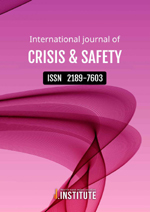상세검색
최근 검색어 전체 삭제
다국어입력
즐겨찾기0KCI등재후보 학술저널

# Crisis & Safety: A Study on the Change of the Urbanization Index according to the Increase or Decrease of Naturalized Plants

• 등재여부 : KCI등재후보
• 2022.03
• 25 - 36 (12 pages)
• 2

Purpose: This study was based on the flora survey data of 1,200 places (776 map index system numbers) over 12 years in the National Natural Environment Survey, Korea s largest basic research project on natural ecosystems. The researchers conducted this study to analyze the differences in the urbanization index by year, altitude, forest land ratio, and population density, investigate the correlations through curvilinear regression analysis, and predict the changes in the urbanization index. Method: The Urbanization Index is the ratio of naturalized plants in the research area to the total number of naturalized plant species (321 species) distributed in South Korea. This paper calculated the urbanization index using the method by Lim Yangjae and Jeon Uisik. One-way ANOVA was performed to analyze the differences in the urbanization index by categories. In addition, curvilinear regression analysis was performed to investigate the correlation between urbanization index and categories. The Spss Statistics 21 statistical program performed the above statistical analyses. Results: The urbanization index showed differences in four categories. In the correlation analysis, the correla-tion between the urbanization index and the years had a positive correlation in which the urbanization index in-creases every year. The urbanization index and the altitudes had a negative correlation in which the urbanization index decreases as the altitude increases. The urbanization index and the forest land ratios had a negative corre-lation in which the urbanization index decreases as the forest land ratio increases. The urbanization index and the population densities had a negative correlation in which the urbanization index decreases as the population density increases, but had a positive correlation in which it increases after a certain point in time. Conclusion: As a result of synthesizing the analyses, the relational formula created from the correlation analy-sis with the years was suitable for predicting the urbanization index. By applying the formula &#119910;=1.754+0.465&#119909;(&#119910;=urbanization index, &#119909;=year), the results of predicting the urbanization index were at 11.01 in 2025, 13.38 in 2030, 18.03 in 2040, and 22.68 in 2050. The urbanization index increased year by year.

1. Introduction

2. Methods

3. Results & Discussion

4. Conclusion

5. References

6. Appendix

로딩중